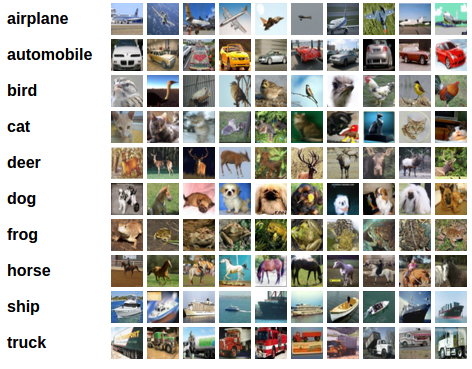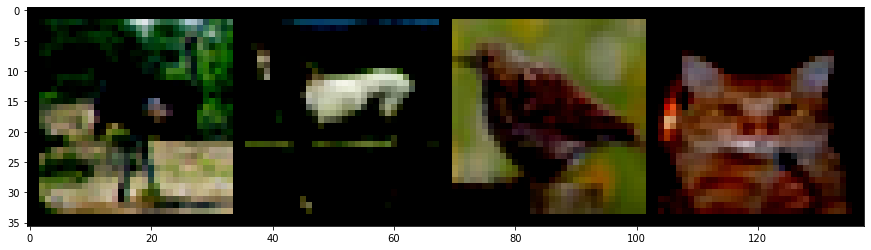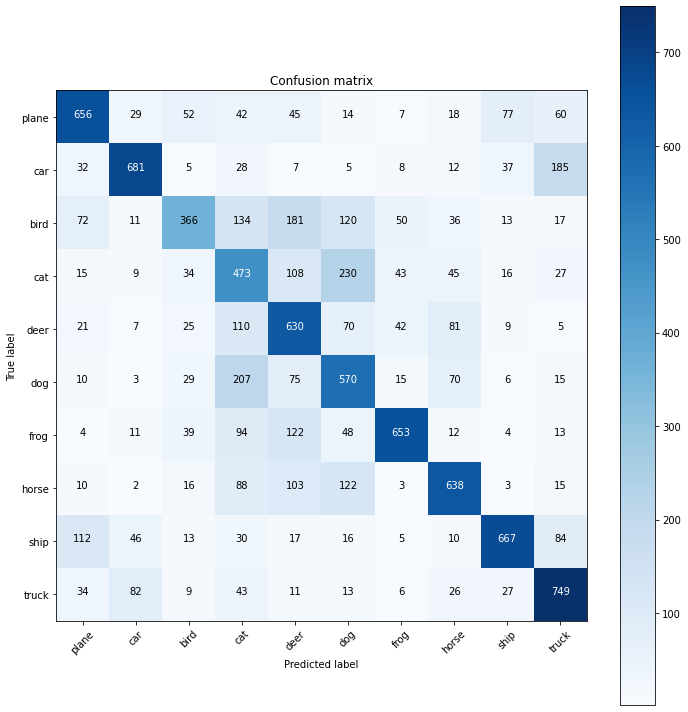For this exercise, we will use the CIFAR10 dataset. It has the classes: ‘airplane’, ‘automobile’, ‘bird’, ‘cat’, ‘deer’, ‘dog’, ‘frog’, ‘horse’, ‘ship’, ‘truck’. The images in CIFAR-10 are of size 3x32x32, i.e. 3-channel color images of 32x32 pixels in size.The dataset is divided into five training batches and one test batch, each with 10000 images. The test batch contains exactly 1000 randomly-selected images from each class. The training batches contain the remaining images in random order, but some training batches may contain more images from one class than another. Between them, the training batches contain exactly 5000 images from each class.

```import torch
import torch.nn as nn
import torch.optim as optim
import torch.nn.functional as F

import torchvision
import torchvision.transforms as transforms

import numpy as np
import matplotlib.pyplot as plt

from sklearn.metrics import confusion_matrix

from torch.utils.tensorboard import SummaryWriter
```
```print(torch.__version__)
print(torchvision.__version__)
```
```1.6.0+cu101
0.7.0+cu101
```

```transform =transforms.Compose([transforms.ToTensor(), transforms.Normalize((0.5, 0.5, 0.5), (0.5, 0.5, 0.5))])

```
```Downloading https://www.cs.toronto.edu/~kriz/cifar-10-python.tar.gz to ./data/cifar-10-python.tar.gz
Extracting ./data/cifar-10-python.tar.gz to ./data
```

Let's view some of the training images in the way they are passed as batches to the Neural Network during the training process.

```batch = next(iter(train_loader))

images, labels = batch

print(images.shape)
print(labels.shape)
grid = torchvision.utils.make_grid(images, nrow=10)

plt.figure(figsize=(15,15))
plt.imshow(grid.permute(1,2,0))

print('labels:', labels)
```
```Clipping input data to the valid range for imshow with RGB data ([0..1] for floats or [0..255] for integers).
```
```torch.Size([4, 3, 32, 32])
torch.Size()
labels: tensor([2, 7, 2, 3])
```## Neural Network and PyTorch design

```class Network(nn.Module):
def __init__(self):
super(Network,self).__init__()
self.conv1 = nn.Conv2d(in_channels=3, out_channels=6, kernel_size=5)
self.conv2 = nn.Conv2d(in_channels=6, out_channels=16, kernel_size=5)

self.fc1 = nn.Linear(in_features=16*5*5, out_features=120)
self.fc2 = nn.Linear(in_features=120, out_features=84)
self.out = nn.Linear(in_features=84, out_features=10)

def forward(self, t):
#Layer 1
t = t
#Layer 2
t = self.conv1(t)
t = F.relu(t)
t = F.max_pool2d(t, kernel_size=2, stride=2)#output shape : (6,14,14)
#Layer 3
t = self.conv2(t)
t = F.relu(t)
t = F.max_pool2d(t, kernel_size=2, stride=2)#output shape : (16,5,5)
#Layer 4
t = t.reshape(-1, 16*5*5)
t = self.fc1(t)
t = F.relu(t)#output shape : (1,120)
#Layer 5
t = self.fc2(t)
t = F.relu(t)#output shape : (1, 84)
#Layer 6/ Output Layer
t = self.out(t)#output shape : (1,10)

return t

network = Network()
```

## Training the Neural Network

```optimizer = optim.Adam(network.parameters(), lr=0.001, betas=(0.9, 0.999), eps=1e-08, weight_decay=0)
```
```for epoch in range(5):

total_correct = 0
total_loss = 0
for batch in train_loader: #Get batch
images, labels = batch #Unpack the batch into images and labels

preds = network(images) #Pass batch
loss = F.cross_entropy(preds, labels) #Calculate Loss

optimizer.step() #Update weights

total_loss += loss.item()
total_correct += preds.argmax(dim=1).eq(labels).sum().item()

print('epoch:', epoch, "total_correct:", total_correct, "loss:", total_loss)

print('>>> Training Complete >>>')
```
```epoch: 0 total_correct: 22538 loss: 18830.44906747341
epoch: 1 total_correct: 28122 loss: 15425.85352185741
epoch: 2 total_correct: 29871 loss: 14222.835543203168
epoch: 3 total_correct: 31315 loss: 13312.894761506468
epoch: 4 total_correct: 32041 loss: 12740.08337423997
>>> Training Complete >>>
```

Let's quickly save the model, we just trained.

```PATH = './cifar_net.pth'
torch.save(network.state_dict(), PATH)
```

## Predictions

We will define a function to get all predictions at once. Now, let's load our Saved Model. Please note that saving and re-loading the model wasn’t necessary here, we only did it to illustrate how to do so.

```network = Network()
```
`<All keys matched successfully>`
```@torch.no_grad()
all_preds = torch.tensor([])
images, labels = batch

preds = model(images)
all_preds = torch.cat((all_preds, preds) ,dim=0)

return all_preds
```
```test_preds = get_all_preds(network, test_loader)
actual_labels = torch.Tensor(test_set.targets)
preds_correct = test_preds.argmax(dim=1).eq(actual_labels).sum().item()

print('total correct:', preds_correct)
print('accuracy:', preds_correct / len(test_set))
```
```total correct: 6083
accuracy: 0.6083
```

The model predicted the label with 61 % accuracy, which is not that great. Next, we will develop a confusion matrix which will demonstrate, in which particular areas our model is performing poorly.

## Confusion Matrix

```import itertools
import numpy as np
import matplotlib.pyplot as plt

def plot_confusion_matrix(cm, classes,
normalize=False,
title='Confusion matrix',
cmap=plt.cm.Blues):
"""
This function prints and plots the confusion matrix.
Normalization can be applied by setting `normalize=True`.
"""
if normalize:
cm = cm.astype('float') / cm.sum(axis=1)[:, np.newaxis]
print("Normalized confusion matrix")
else:
print('Confusion matrix, without normalization')

print(cm)

plt.imshow(cm, interpolation='nearest', cmap=cmap)
plt.title(title)
plt.colorbar()
tick_marks = np.arange(len(classes))
plt.xticks(tick_marks, classes, rotation=45)
plt.yticks(tick_marks, classes)

fmt = '.2f' if normalize else 'd'
thresh = cm.max() / 2.
for i, j in itertools.product(range(cm.shape), range(cm.shape)):
plt.text(j, i, format(cm[i, j], fmt),
horizontalalignment="center",
color="white" if cm[i, j] > thresh else "black")

plt.tight_layout()
plt.ylabel('True label')
plt.xlabel('Predicted label')
```
```cm = confusion_matrix(test_set.targets, test_preds.argmax(dim=1))
classes = ('plane', 'car', 'bird', 'cat', 'deer', 'dog', 'frog', 'horse', 'ship', 'truck')
plt.figure(figsize=(10,10))
plot_confusion_matrix(cm, classes)
```
```Confusion matrix, without normalization
[[656  29  52  42  45  14   7  18  77  60]
[ 32 681   5  28   7   5   8  12  37 185]
[ 72  11 366 134 181 120  50  36  13  17]
[ 15   9  34 473 108 230  43  45  16  27]
[ 21   7  25 110 630  70  42  81   9   5]
[ 10   3  29 207  75 570  15  70   6  15]
[  4  11  39  94 122  48 653  12   4  13]
[ 10   2  16  88 103 122   3 638   3  15]
[112  46  13  30  17  16   5  10 667  84]
[ 34  82   9  43  11  13   6  26  27 749]]
```